# 5. Experimental Results

## 5. Experimental Results

In this section we are going to discuss and evaluate the experimental results of applying color anglogram and latent semantic indexing to video shot detection. We will also compare the performance of these methods with that of some existing shot detection techniques. Our data set consisted of 8 video clips of which the total length is 496 seconds. A total of 255 abrupt shot transitions (cuts) and 60 gradual shot transitions were identified in these clips. Almost all of the possible editing effects, such as cuts, fades, wipes, and dissolves, can be found in these clips. These clips contain a variety of categories ranging from outdoor scenes and news story to TV commercials and movie trailers. All these video clips were converted into AVI format using a software decoder/encoder. A sample clip (outdoor scene) is presented in Figures 15.4 and 15.5. Figure 15.4 shows the 4 abrupt transitions and Figure 15.5 shows the gradual transition.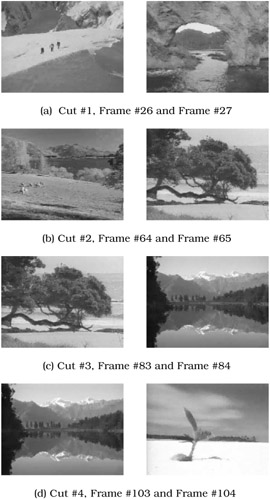Figure 15.4: Abrupt Shot Transitions of a Sample Video Clip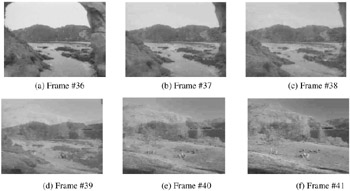Figure 15.5: Gradual Shot Transition of a Sample Video Clip

Our shot detection evaluation platform is shown in Figure 15.6. This system supports video playback and frame-by-frame browsing and provides a friendly interface. It allows us to compare the performance of various shot detection techniques, such as pair-wise comparison, global and local color histogram, color histogram with x2, color anglogram, and latent semantic indexing, and a combination of these techniques. Several parameters, such as number of blocks, number of frames, and various thresholds used in feature extraction and similarity comparison, can be adjusted. Statistical results of both abrupt transitions and gradual transitions, together with their locations and strength, are presented in both chart and list format. The results of using various shot detection methods on a sample video clip are shown in Figure 15.7. Our evaluation platform also measures the computational cost of each shot detection process in terms of processing time.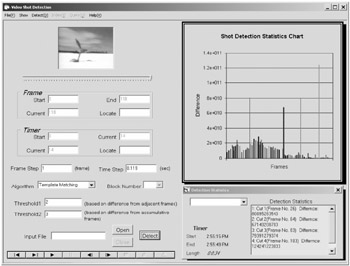Figure 15.6: Video Shot Detection Evaluation System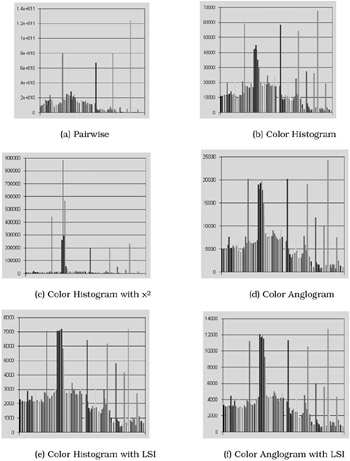Figure 15.7: Shot Detection Result of a Sample Video Clip

Two thresholds, T1 and T2, are involved in the similarity comparison process, which are similar to those in [20, 37]. If the distance between two consecutive frames fi and fi+1 is above T1, a shot transition is identified between frames fi and fi+1. If the distance between fi and fi+1 is below T2, the two frames are considered to be within the same shot. If the distance falls in the range between these two thresholds, further examination will be necessary to determine if the distance results from a gradual transition or not. A certain number of frames, N, can be specified by the user, which allows the system to analyze the accumulative similarity of frames fi-N, fi-N+1, .,.and fi. This measure will be compared with frame fi+1 and thus to determine if a transition exists between fi and fi+1.

In our experiment, we compared the performance of our color anglogram approach with that of the color histogram method, due to the fact that color histogram provides one of the best performance among existing techniques. Then, we applied the latent semantic indexing technique to both color histogram and color anglogram, and evaluated their shot detection performance. The complete process of visual feature extraction and similarity comparison is outlined as follows.

Each frame is converted into the HSV color space. For each pixel of the frame, hue and saturation are extracted and each quantized into a 10-bin histogram. Then, the two histograms h and s are combined into one h s histogram with 100 bins, which is the representing feature vector of each frame. This is a vector of 100 elements, F = [f1, f2, f3, ...f100]T.

To apply the latent semantic indexing technique, a feature-frame matrix, A = [F1,.,. Fn], where n is the total number of frames of a video clip, is constructed using the feature vector of each frame. Each row corresponds to one of the feature elements and each column is the entire feature vector of the corresponding frame.

Singular Value Decomposition is performed on the feature-frame matrix. The result comprises three matrices, U, , and V, where A = UVT. The dimensions of U, , and V are 100 100, 100 n, and n n, respectively. For our data set, the total number of frames of each clip, n, is greater than 100. To reduce the dimensionality of the transformed space, we use a rank-k approximation, Ak, of the matrix A, where k = 12. This is defined by Ak = UkkVkT. The dimension of Ak is the same as A, 100 by n. The dimensions of Uk, k, and Vk are 100 12, 12 12, and n 12, respectively.

The following normalization process will assign equal emphasis to each frame of the feature vector. Different components within the vector may be of totally different physical quantities. Therefore, their magnitudes may vary drastically and thus bias the similarity measurement significantly. One component may overshadow the others just because its magnitude is relatively too large. For the feature-frame matrix A=[V1,V2, .,.V n], we have Ai,j which is the ith component in vector Vj. Assuming a Gaussian distribution, we can obtain the mean, μi, and standard deviation, σi, for the ith component of the feature vector across all the frames. Then we normalize the original feature-frame matrix into the range of [-1,1] as follows,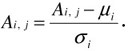It can easily be shown that the probability of an entry falling into the range of [-1, 1] is 68%. In practice, we map all the entries into the range of [-1, 1] by forcing the out-of-range values to be either -1 or 1. We then shift the entries into the range of [0, 1] by using the following formulaAfter this normalization process, each component of the feature-frame matrix is a value between 0 and 1, and thus will not bias the importance of any component in the computation of similarity.

One of the common and effective methods for improving full-text retrieval performance is to apply different weights to different components . We apply these techniques to our experiment. The raw frequency in each component of the feature-frame matrix, with or without normalization, can be weighted in a variety of ways. Both global weight and local weight are considered in our approach. A global weight indicates the overall importance of that component in the feature vector across all the frames. Therefore, the same global weighting is applied to an entire row of the matrix. A local weight is applied to each element indicating the relative importance of the component within its vector. The value for any component Ai, j is thus L(i, j)G(i), where L(i, j) is the local weighting for feature component i in frame j, and G(i) is the global weighting for that component.

Common local weighting techniques include term frequency, binary, and log of term frequency, whereas common global weighting methods include normal, gfidf, idf, and entropy. Based on previous research, it has been found that log (1 + term frequency) helps to dampen effects of large differences in frequency and thus has the best performance as a local weight, whereas entropy is the appropriate method for global weighting .

The entropy method is defined by having a component global weight of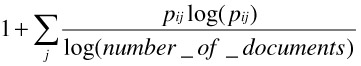where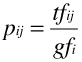is the probability of that component, tfij is the raw frequency of component Ai,j, and gfi is the global frequency, i.e., the total number of times that component i occurs in all the frames.

The global weights give less emphasis to those components that occur frequently or in many frames. Theoretically, the entropy method is the most sophisticated weighting scheme, taking the distribution property of feature components over the set of all the frames into account.

We applied color histogram to shot detection and evaluated the results of using it with and without latent semantic indexing. The experimental results are presented in Table 15.1. The measures of recall and precision are used in evaluating the shot detection performance. Consider an information request I and its set R of relevant documents. Let |R| be the number of documents in this set. Assume that a given retrieval method generates a document answer set A and let |A| be the number of documents in this set. Also, let |Ra| be the number of documents in the intersection of the sets R and A. Then recall is defined as

Table 15.1: Evaluations of Experimental Results

Abrupt Shot Transition

Gradual Shot Transition

Precision

Recall

Precision

Recall

Color Histogram

70.6%

82.7%

62.5%

75.0%

Color Histogram with LSI

74.9%

83.1%

65.8%

80.0%

Color Anglogram

76.5%

88.2%

69.0%

81.7%

Color Anglogram with LSI

82.9%

91.4%

72.6%

88.3%

Recall = |Ra| / |R|

which is the fraction of the relevant documents that has been retrieved, and precision is defined as

Precision = |Ra| / |A|

which is the fraction of the retrieved documents that are considered as relevant. It can be noticed that better performance is achieved by integrating color histogram with latent semantic indexing. This validates our beliefs that LSI can help discover the correlation between visual features and higher level concepts, and thus help uncover the semantic correlation between frames within the same shot.

For our experiments with color anglogram, we still use the hue and saturation values in the HSV color space, as what we did in the color histogram experiments. We divide each frame into 64 blocks and compute the average hue value and average saturation value of each block. The average hue values are quantized into 10 bins; so are the average saturation values. Therefore, for each quantized hue (saturation) value, we can apply Delaunay triangulation on the point feature map. We count the two largest angles of each triangle in the triangulation, and categorize them into a number of anglogram bins each of which is 5 . Our vector representation of a frame thus has 720 elements: 36 bins for each of the 10 hue values and 36 bins for each of the 10 saturation values. In this case, for each video clip the dimension of its feature-frame matrix is 720 n, where n is the total number of frames. As is discussed above, we reduce the dimensionality of the feature-frame matrix to k = 12. Based on the experimental results of our previous studies in [38, 39], we notice that normalization and weighting has a negative impact on the performance of similarity comparison using color anglogram. Therefore, we do not apply normalization and weighting on the elements in the feature-frame matrix.

We compare the shot detection performance of using color anglogram with or without latent semantic indexing, and the results are shown in Table 15.1. From the results we notice that the color anglogram method achieves better performance than color histogram in capturing meaningful visual features. This result is consistent with those of our previous studies in [33, 34, 38, 39, 40]. One also notices that the best performance of both recall and precision is provided by integrating color anglogram with latent semantic indexing. Once again our experiments validated that using latent semantic indexing to uncover the semantic correlations is a promising approach to improve content-based retrieval and classification of image/video documents.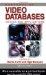Handbook of Video Databases: Design and Applications (Internet and Communications)
ISBN: 084937006X
EAN: 2147483647
Year: 2003
Pages: 393

flylib.com © 2008-2017.
If you may any questions please contact us: flylib@qtcs.net